# CS 61B | Part 11 | Topological Sort, DAG-LPT, DAG-SPT, DP, LIS / LLIS

## Topological Sort

### Definition

Suppose we have a collection of different tasks or activities, some of which must happen before another. How do we find the way to sort the tasks such that for each task $v$, the tasks that happen before $v$ come earlier in our sort?

In the graph, each node represents a task. An edge $v\rightarrow w$ indicates that $v$ must happen before $w$. Now our original problem is reduced to finding a topological sort.

Topological Sort: An ordering of a graph’s vertices such that for every directed edge $u \rightarrow v$, $u$ comes before $v$ in the ordering.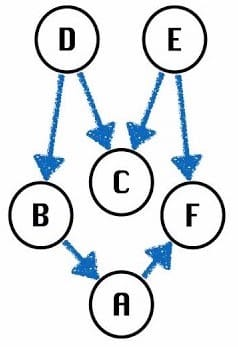Valid orderings including:

• D, B, A, E, C ,F
• E, D, C, B, A, F

Note: If I purely run DFS, the output is D, B, A, F, E, C, E, F. It is not correct.

Certain Types of Graphs (DAG):

Also, it only makes sense to topological sort some certain types of graphs. Here is an invalid example: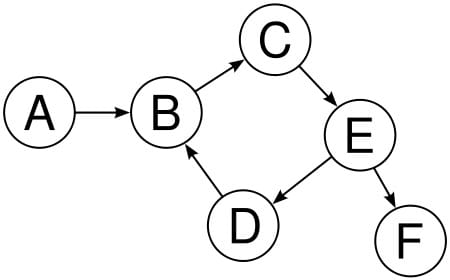Since we have a cycle, topological sort is not defined. Also, it includes undirected graphs (each edge corresponds to a cycle):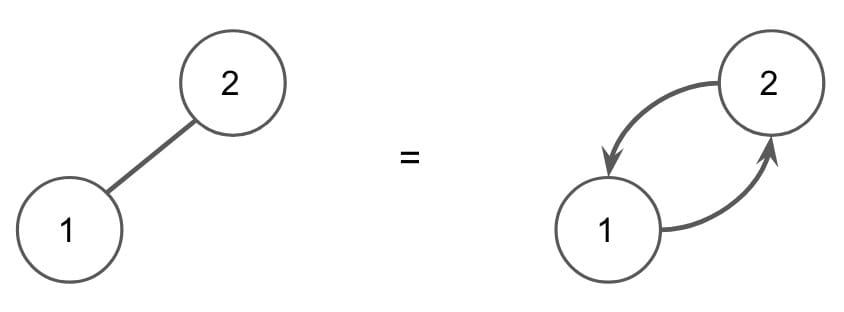All in all, topological sorts only apply to directed, acyclic graphs, or DAGs.

DAG (/dag/) is a very important type of graphs.

Topological Sort: An ordering of DAG‘s vertices such that for every directed edge $u \rightarrow v$, $u$ comes before $v$ in the ordering. Each DAG corresponds to a Topological Sort.

For any topological ordering, you can redraw the graph so that the vertices are all in one line. Thus, topological sort is sometimes called a linearization of the graph.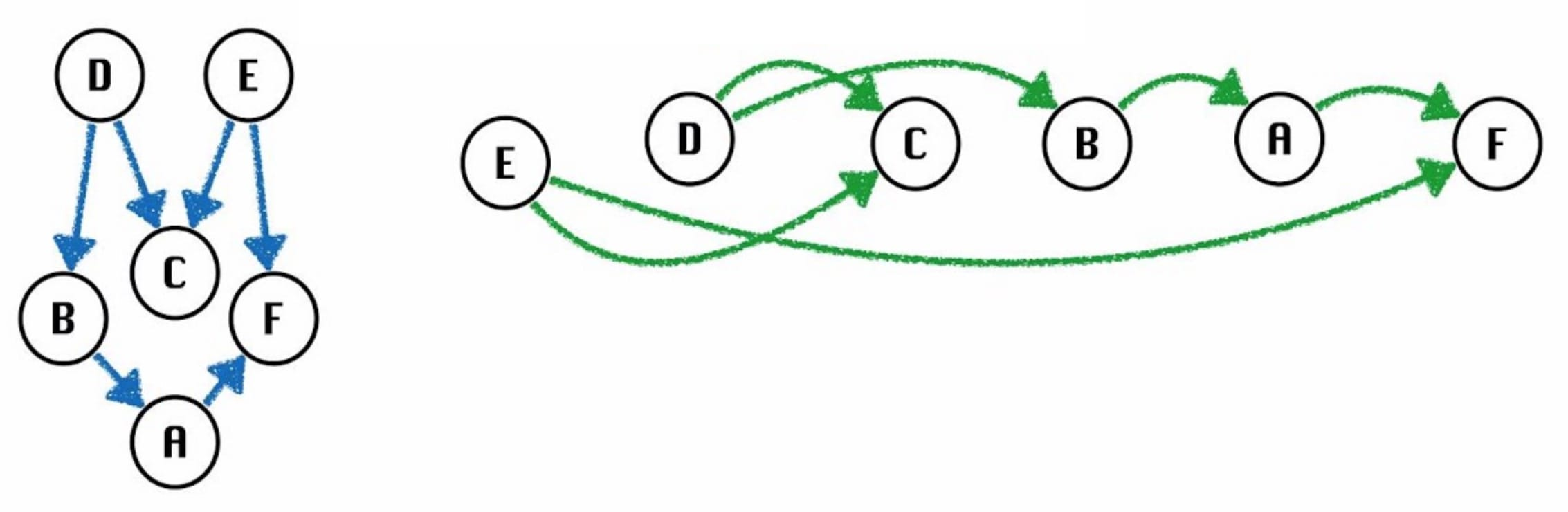In the above DAG, $D$ or $E$ must be the source, $F$ or $C$ must be the end, also called sink.

### TS Algorithm

Topological Sort Algorithm:

1. Perform a DFS traversal from every vertex (any order) in the graph, not clearing markings between traversals (means not traversing marked vertices).
2. Record DFS post-order along the way (add to list when backtracking).
3. Topological ordering is the reverse of the post-order.If we use DFS only, the ordering is wrong. For example:

• D, C, B, A, F, E (C comes before E)

Why it works:

Each vertex $v$ gets added to the end of the post-order list only after considering all descendants of $v$, which means all of them are already on the list. For example, if I want to add vertex $D$, it happens only after I’ve added $C$ and other descendants ($B$, $A$, $F$) to the list. It is guaranteed in the process.

Runtime: $O(V + E)$

### Pseudocode

Using BFS:

Reference: Using BFS for topological sort

How to calculate the 0-in-degree vertices from all vertices?

• Adj Matrix: Foreach columns and store 0-in-degree vertices.
• Adj List & Edge List: Foreach all vertices, count, and store 0-in-degree vertices.

Or, we can modify it a little bit (change the output ordering of zero in-degree vertices):

Alternative (From GitBook):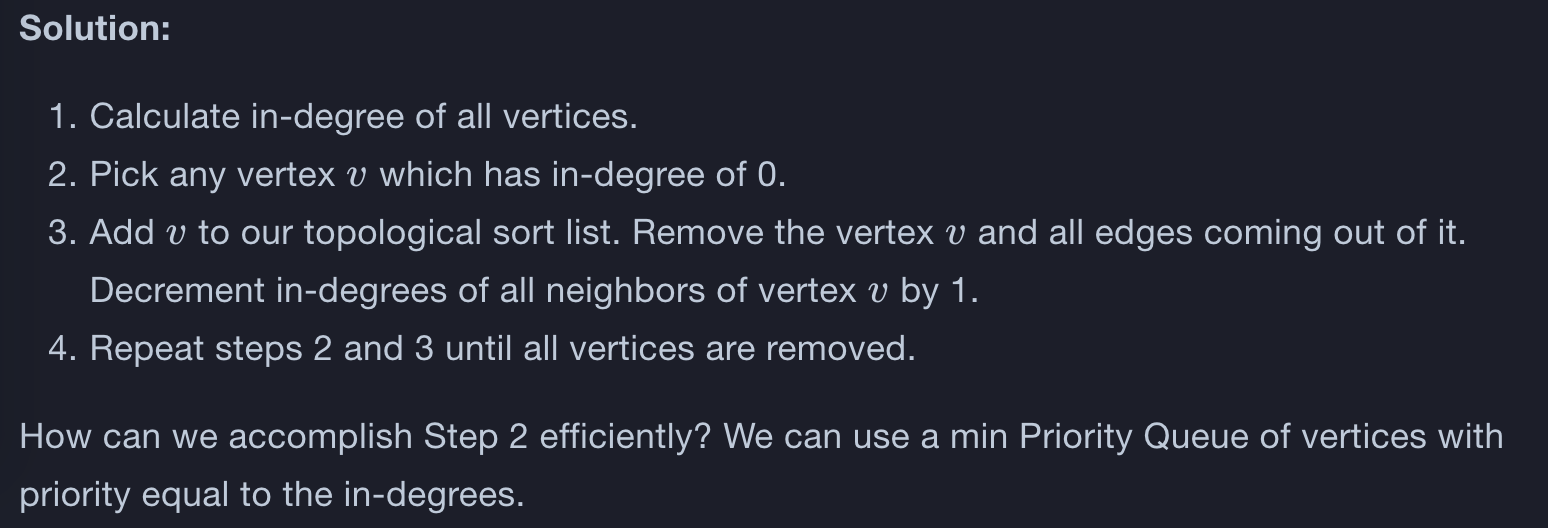## DAG-LPT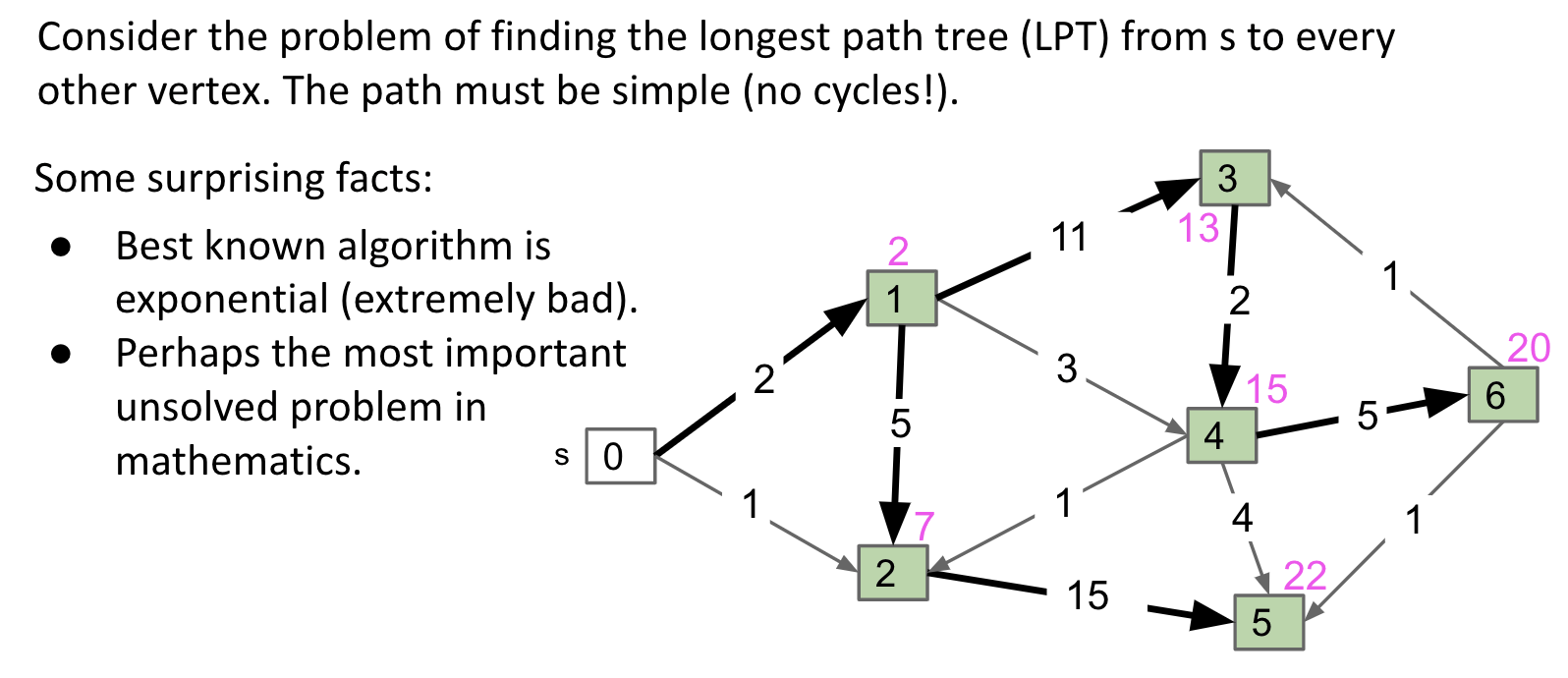Consider the longest paths problem on DAGs:

• From a new copy of the graph $G’$ with signs of all edge weights flipped.
• Run DAG-SPT on $G’$ yielding result $X$.
• Flip signs of all values in $X$.distTo.
• $X$.edgeTo is already correct.

There is no real need to prove anything or show demos.

• We know DAG-SPT works on graphs with negative edge weights to get the shortest paths.
• Assuming weights are $w_1, w_2, \ldots, w_E$,
we know that $-(-a + -b + -c + -d) = a + b + c + d$.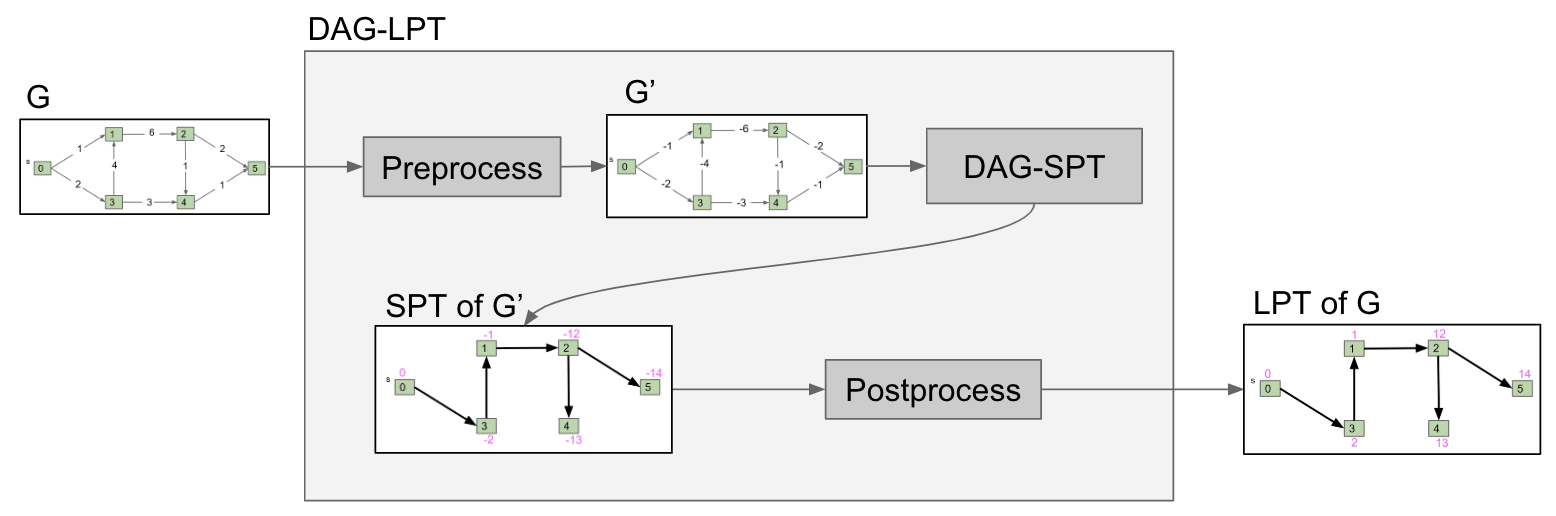## DAG-SPT

In a DAG, we can use Dijkstra’s algorithm to find the SPT. It’s simpler: DAG-SPT Algorithm.

One Simple Idea: Visit vertices in topological order.

• When we visit a vertex: Relax all of its going edges.
• Each vertex is visited only when all possible info about it has been used.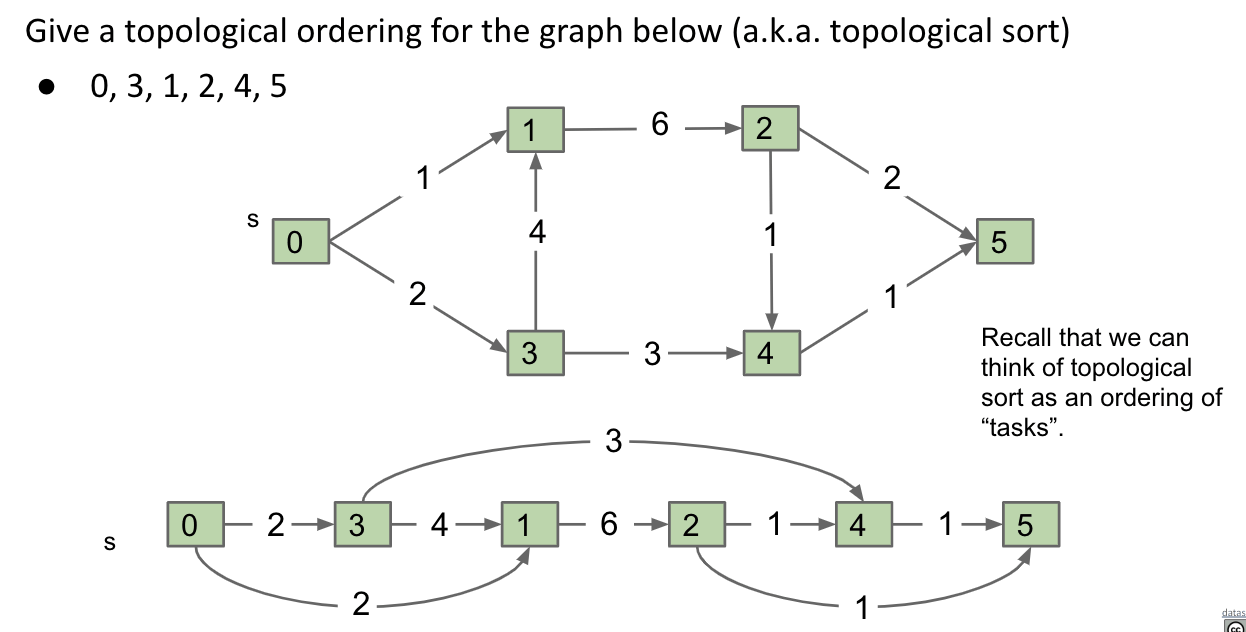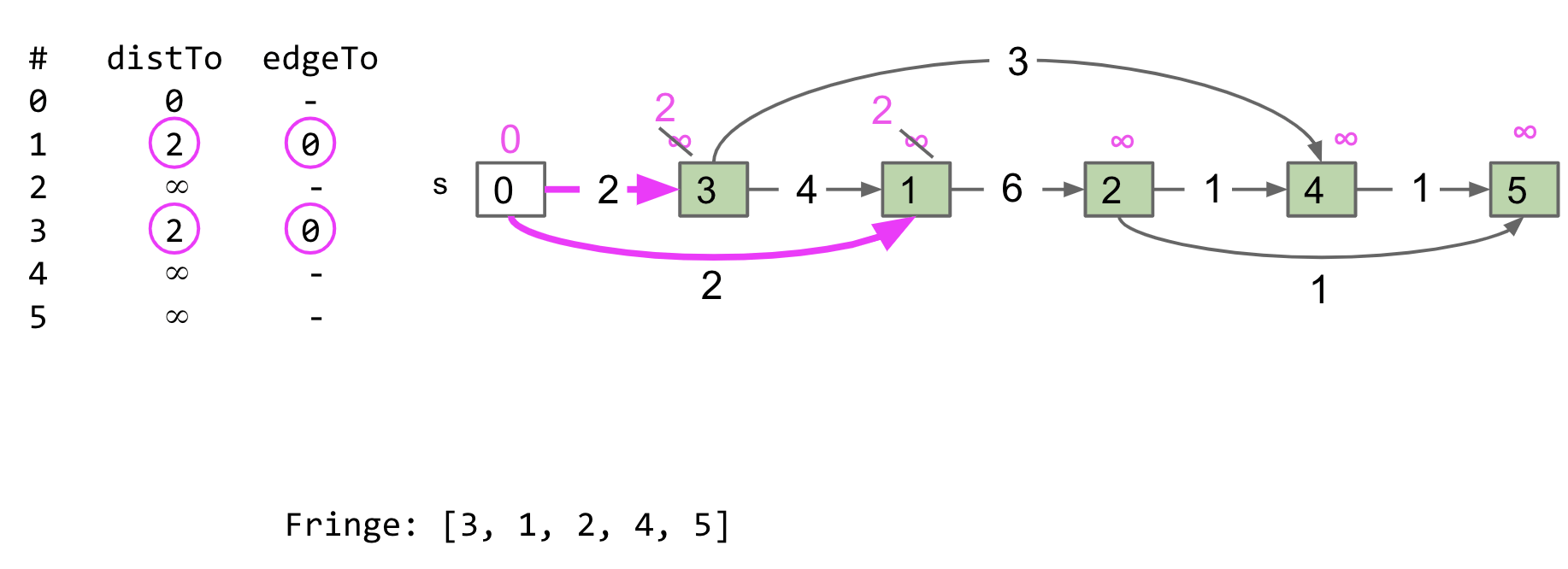Note: DAG-SPT can solve SPT in DAGs with negative weights.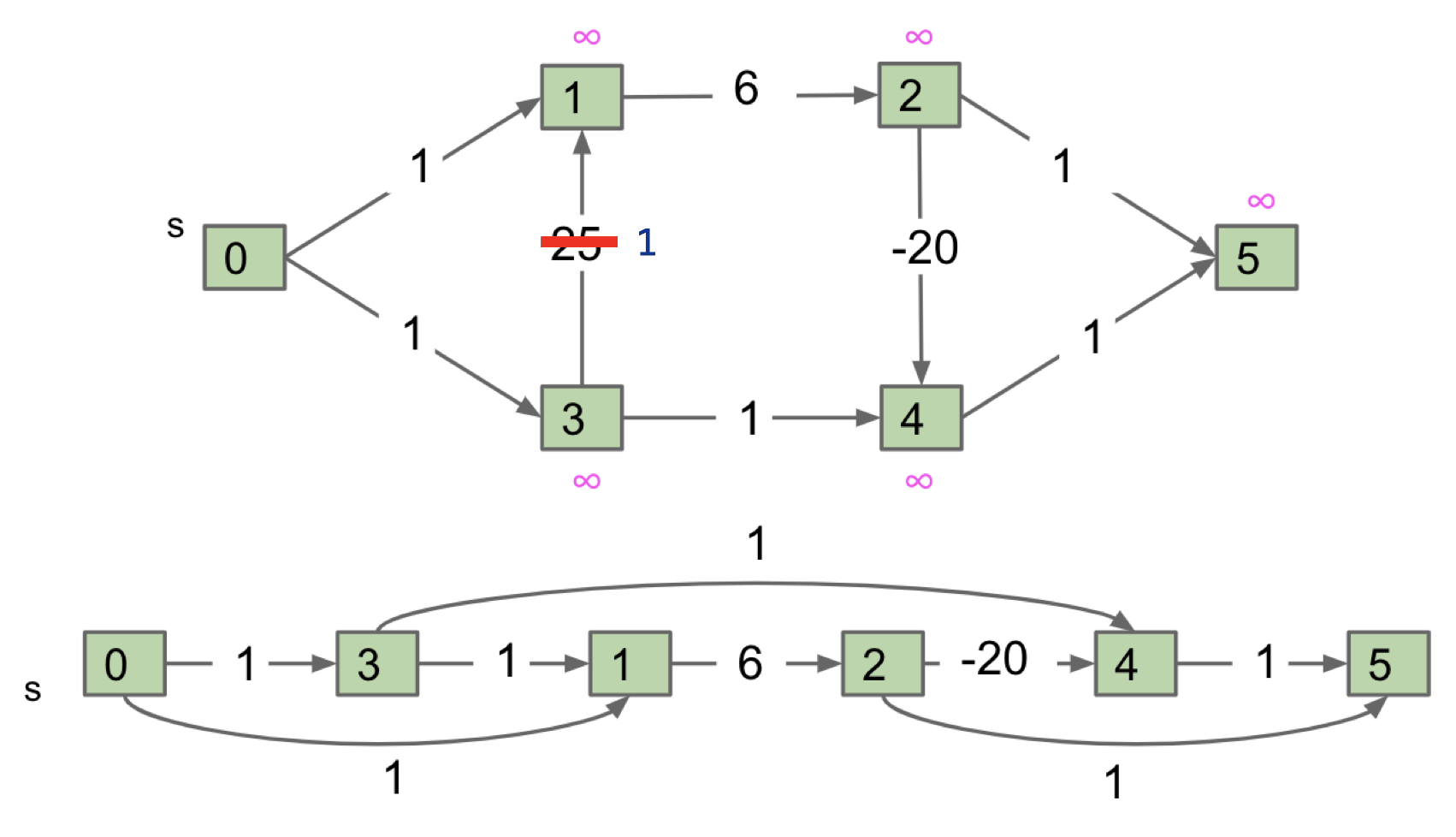Dijkstra’s can’t handle the negative weight and the result is incorrect.

Runtime: $\Theta(E + V)$

• Step 1: Have to do a topological sort, which is $\Theta(E + V)$ time.
• Step 2: Initialize some arrays of size $V$. In total, takes $\Theta(V)$ time.
• Step 3: Each edge gets relaxed exactly once, so $\Theta(E)$.

DP In DAG:

The DAG-SPT algorithm can be thought of as solving increasingly large subproblems:

• Distance from source to source is very easy, and is just zero.
• We then tackle distances to vertices that are a bit farther to the right.
• We repeat this until we get all the way to the end of the graph.

## Dynamic Programming

Dynamic Programming is a terrible name for a simple and powerful idea for solving “big problems”.

• Identify a collection of subproblems.
• Solve subproblems one by one, working from smallest to largest.
• Use the answers to the smaller problems to help solve the larger ones.

by design = as a result of a plan; intentionally

## LIS / LLIS

Problem: Given a sequence of numbers, find the longest increasing subsequence (LIS).

Example:

• Sequence: 6, 2, 8, 4, 5, 7
• The LIS: 2, 4, 5, 7 (LLIS = 4)

Related Problem: Find the length of the longest increasing subsequence (LLIS).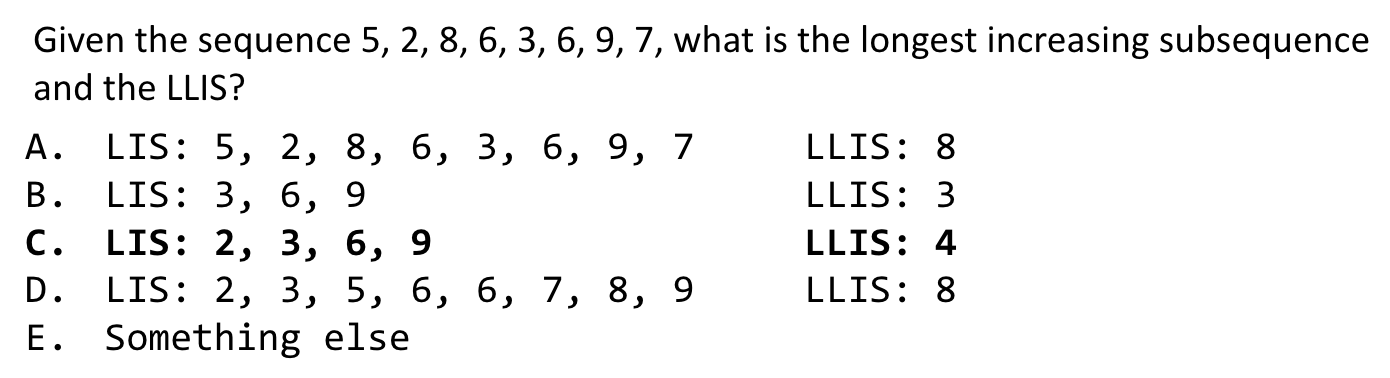### LLIS & DAG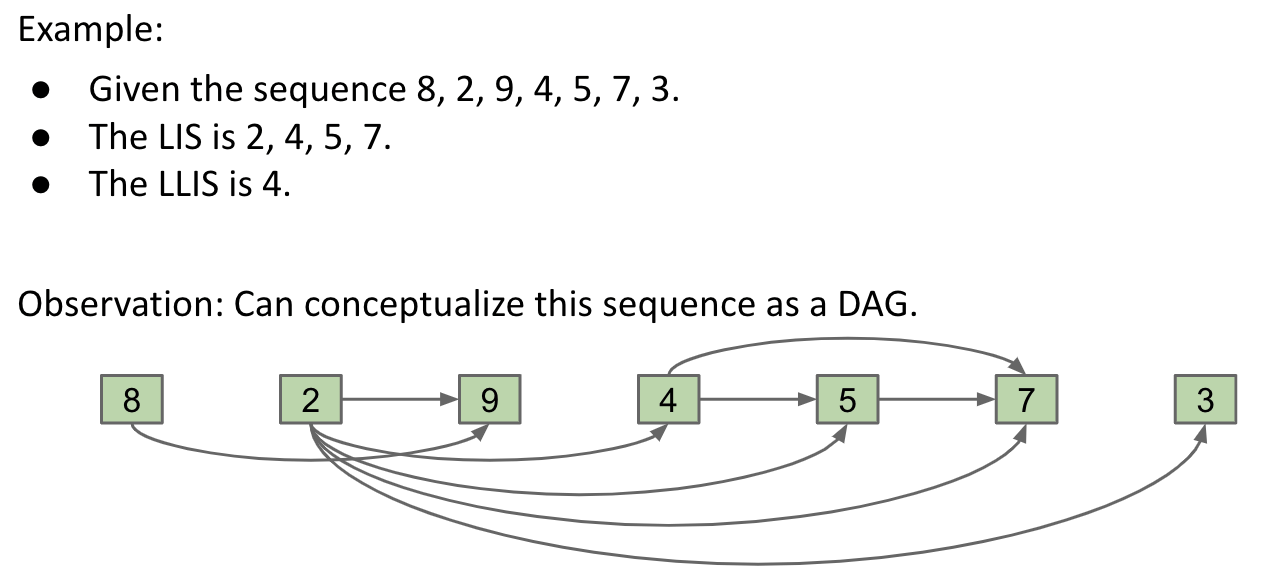#### Conversion (DAG)

Goal: Describe the LLIS problem in terms of a DAG problem. What property of the DAG are we looking for?

Property: The longest path from any vertex to any vertex in the entire DAG (actually plus one).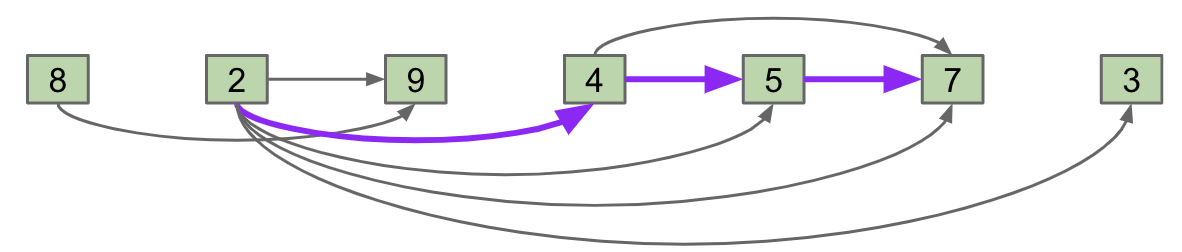So our goal is boiled down to design an algorithm for finding the length of the longest path in a DAG.

#### Clever Idea (-1)

Clever Idea: Using a Negative Weight Graph to Find Longest paths.

• Create copy with edge weights set to $-1$. ==> $\Theta(E+V)$ (applied to adjacency list)
• For each vertex $v$: ==> $V$ times
• Run DAG-SPT Algorithm from $v$ to the end. (Recall this is a DP algorithm) ==> $\Theta(E+V)$
• Stops when a vertex is not reachable from $v$.
• Let LPLS[v] = abs(min(distTo)), i.e. length of the longest path starting at $v$.
• Each time you need to reset distTo and edgeTo.
• Return max(LPLS). ==> $\Theta(V)$

In the worst case, total runtime is $\Theta(EV + V^2)$.

Note: In the worst case:

• $\Theta(N) = \Theta(V)$

• $\Theta(E) = \Theta(V^2) = \Theta(N^2)$. (Each vertex connects other vertices)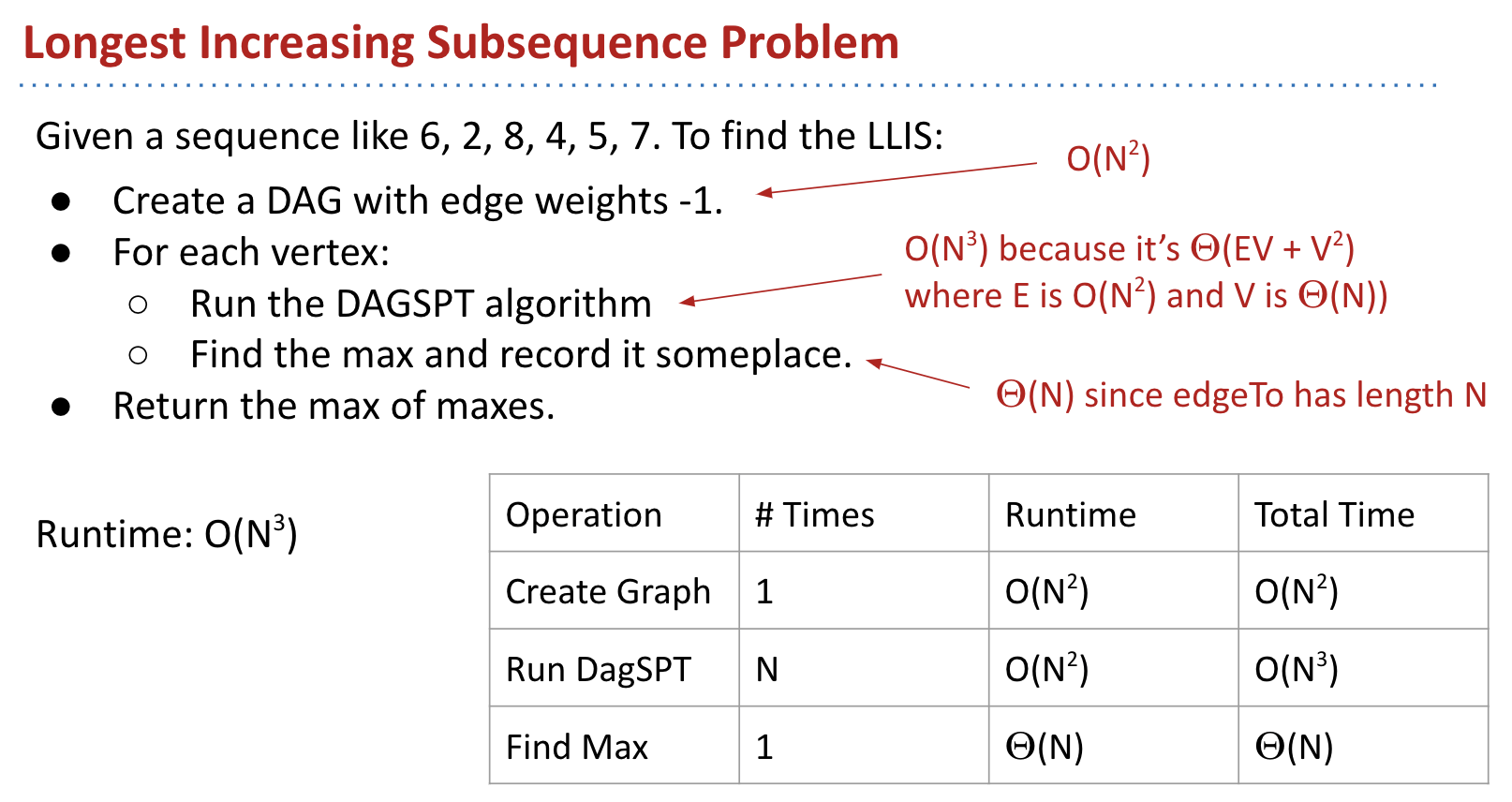#### Demo Example

Initialized all edges with $-1$: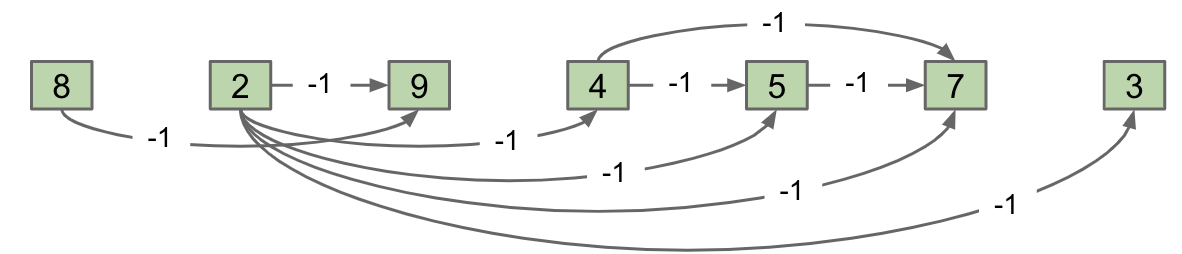DAG-SPT with s = 8: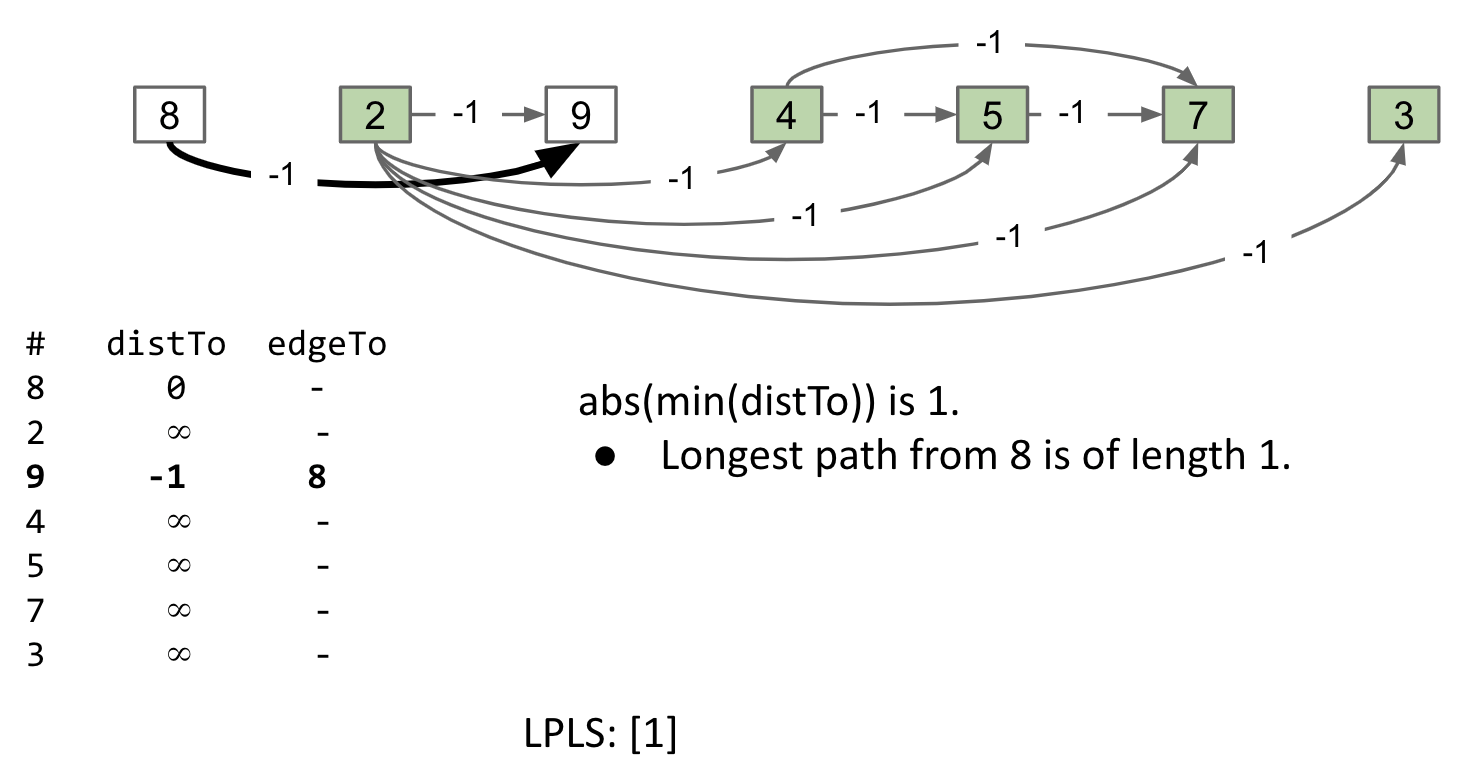Note: Stops at vertex 9, since vertex 2 is not reachable from vertex 8.

DAG-SPT with s = 2: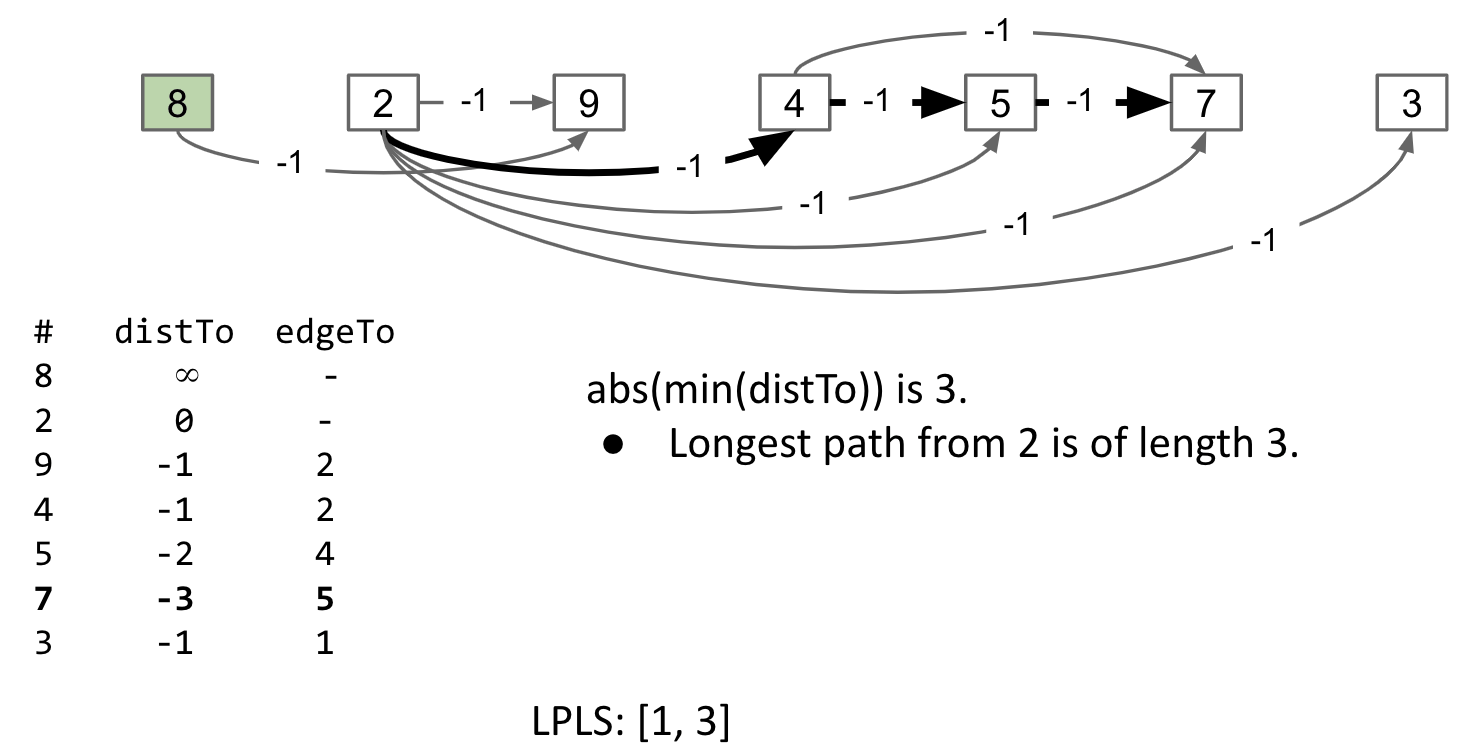Note: Go through all vertices since vertex 9, 4, 5, 7, 3 are all reachable.

The final state of the algorithm: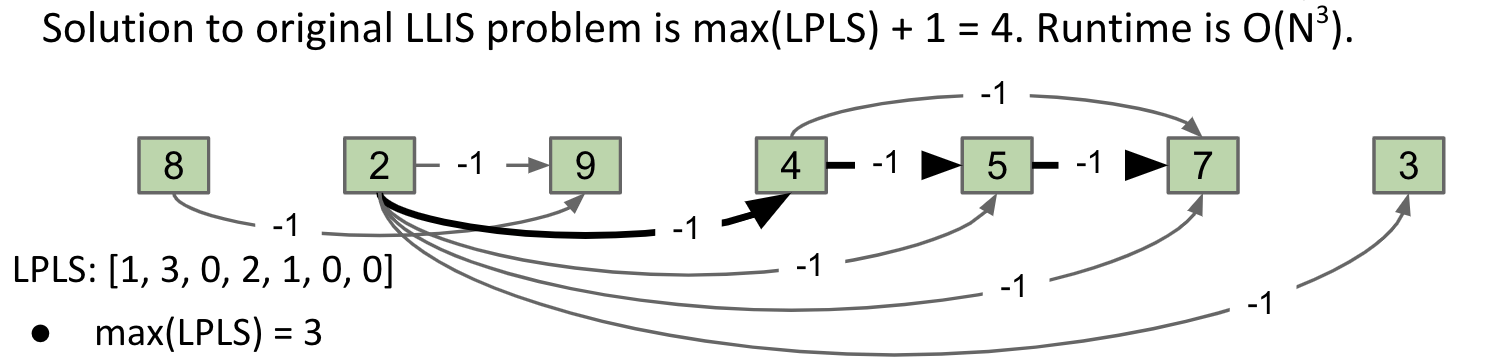#### Reduction

Given a sequence of integers, we transformed our problem into a problem on DAGs. This process is called reduction. We “reduced” LLIS to $N$ solutions of the longest paths problem on DAGs.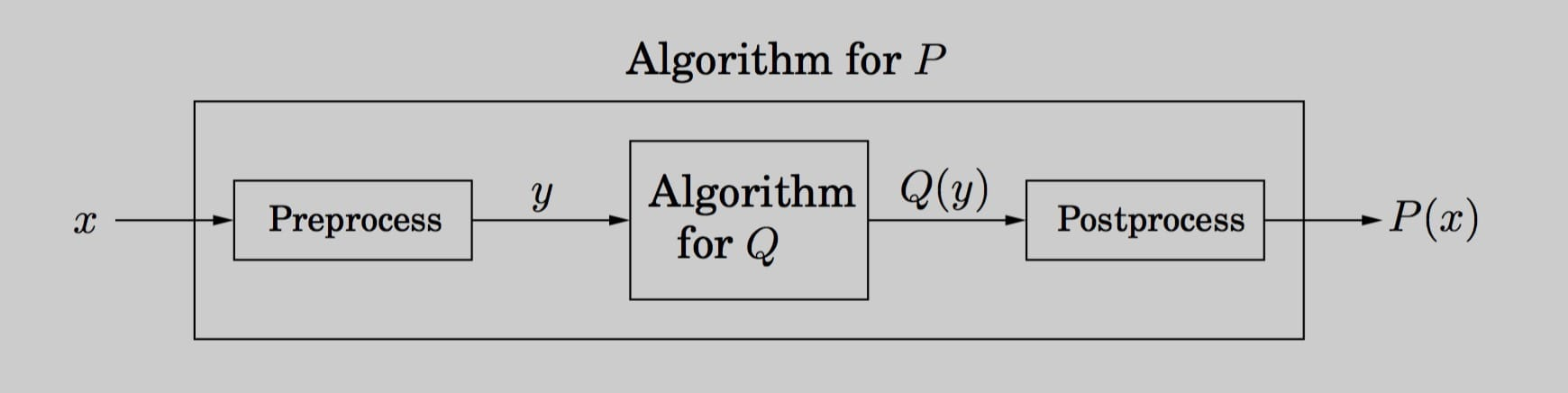Other informal examples:

• Reduce illuminating a room to flipping a light switch.
• Reduce getting to work to riding BART.
• Reduce 8puzzle to A*.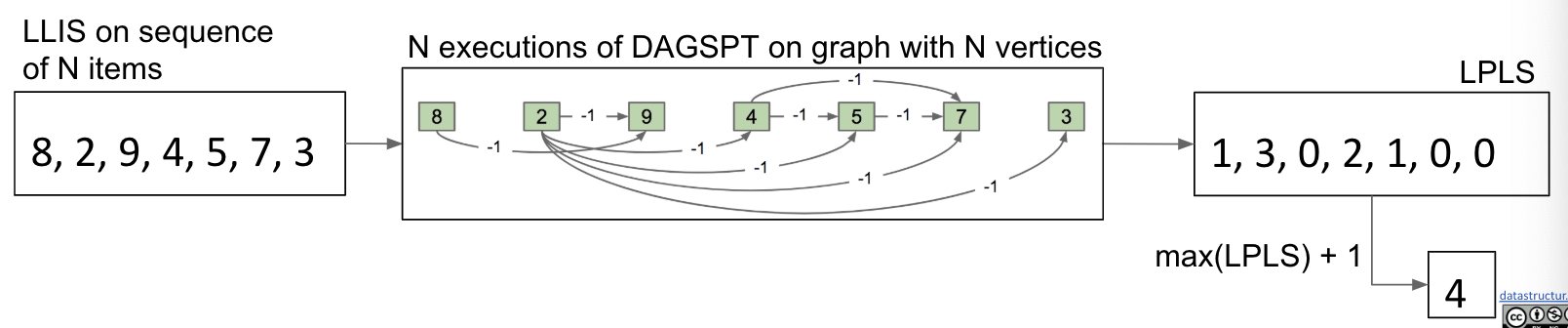### Using DP

#### Redundancy

In effect, we are solving a bunch of subproblems in the wrong order.

The process we described was redundant. Example: DAG-SPT for s = 4 is a subproblem of DAG-SPT for s = 2, but we ran both in their entirety.

#### New Subproblem

Usually, finding a “right” subproblem of DP is very hard.

Rather than choosing where it starts, we can choose the following subproblem for LLIS:

• Length of the Longest Subsequence Ending At (LLSEA)

#### Examples

Let’s see some examples.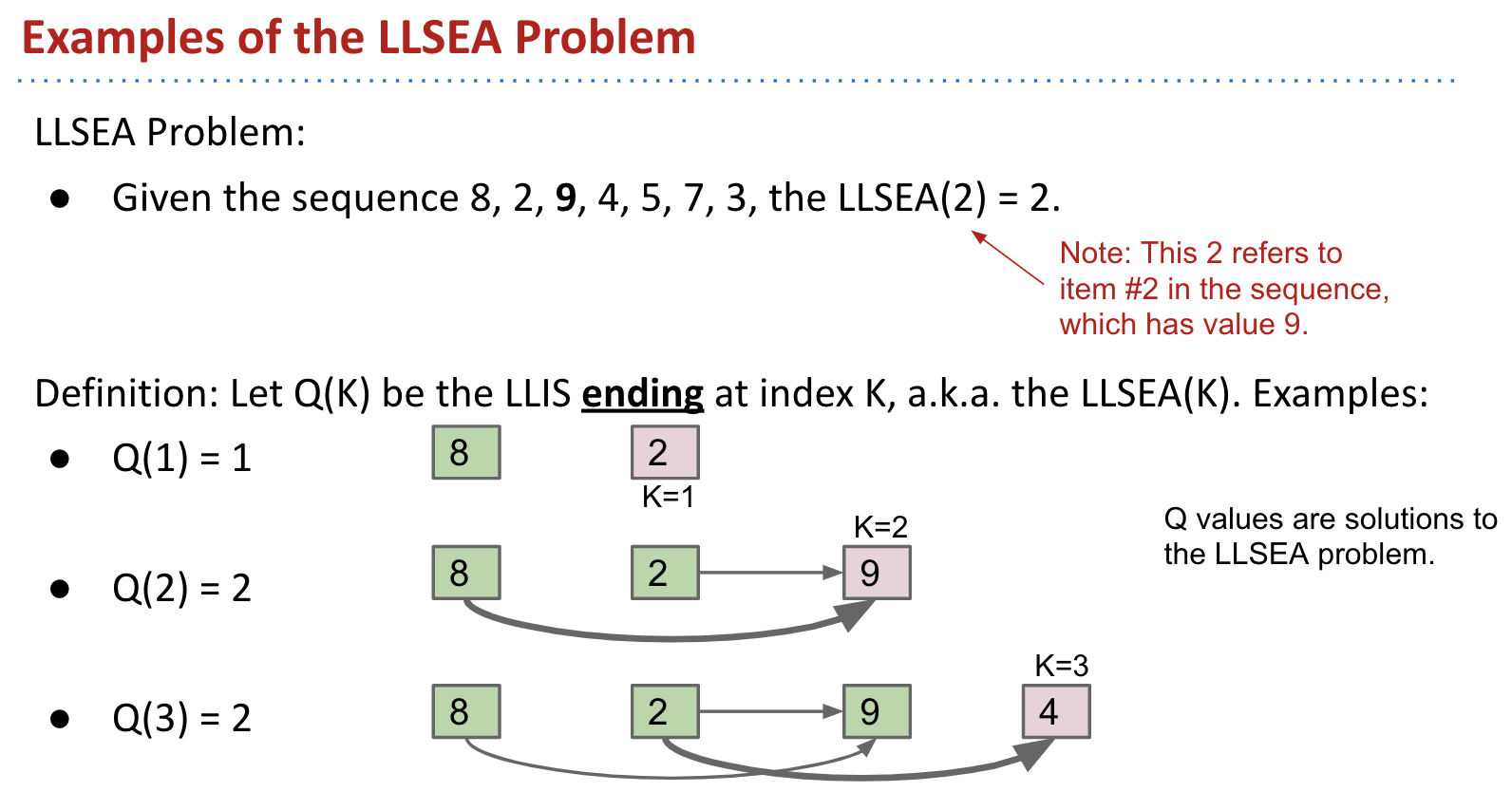$Q(2) = \text{max}(Q(0) + 1, Q(1) + 1) = 2$
$Q(3) = Q(1) + 1 = 2$

Recall that $Q(K)$ is the solution to LLSEA(K) (they are equivalent).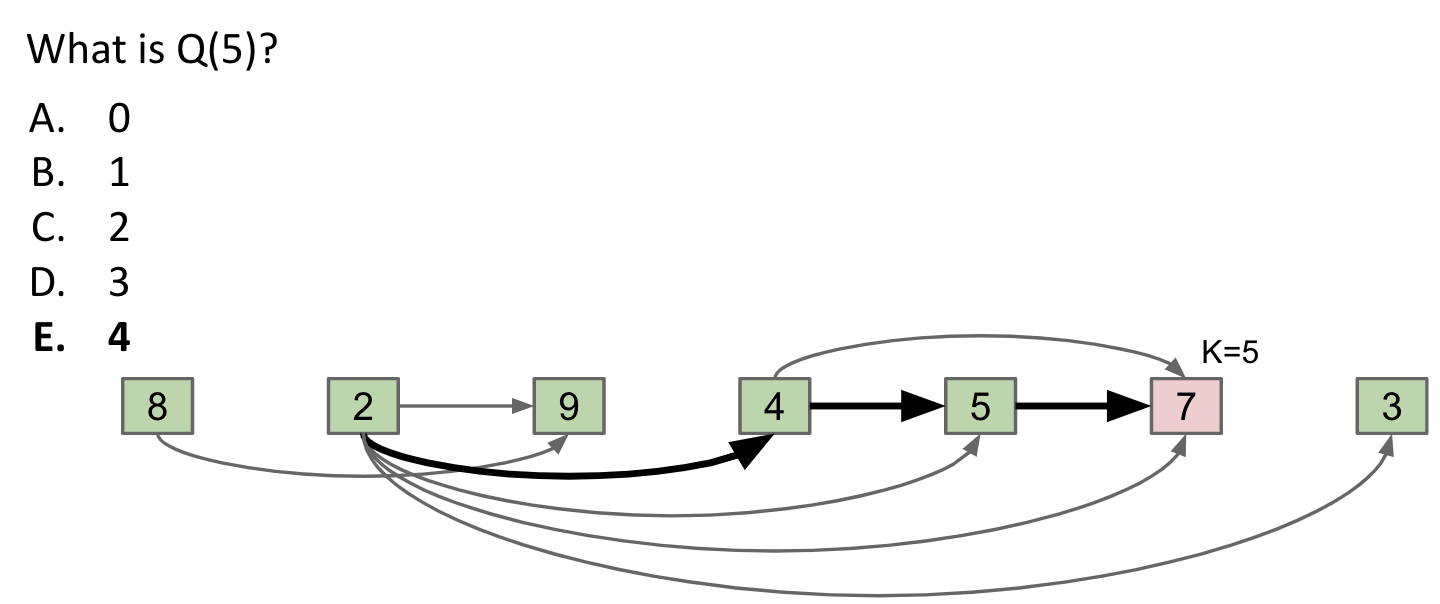$Q(5) = \text{max}{ Q(1) + 1, Q(3) + 1, Q(4) + 1 } = Q(5) + 1 = 4$

#### Fact #1: Memo

Fact #1: We can use results for small $Q$ to compute results for larger $Q$.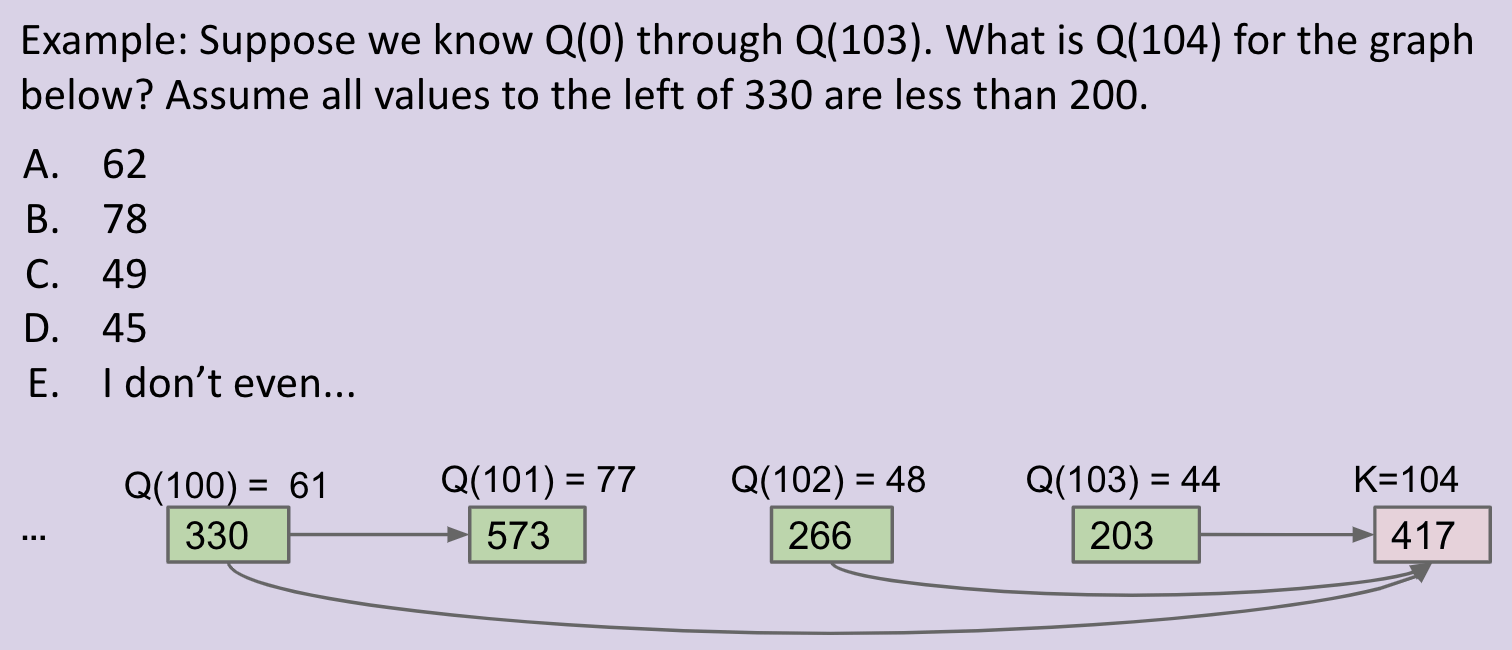The answer is $62$. Because of all the increasing subsequences that have an edge pointing to vertex 104, the longest one was of length $61$ (ending at vertex 100).

The $Q$ values are memorized answers to smaller subproblems.

• The LLIS ending at vertex 417 is the max of $1 + Q(100)$.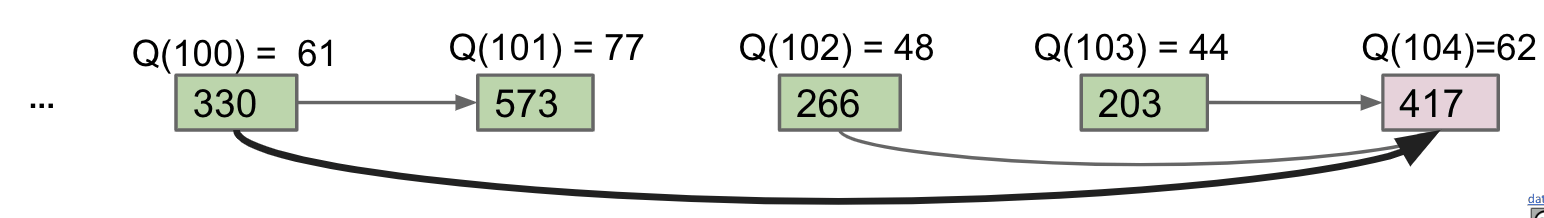#### Fact #2: Solvable

Fact #2: We can use our $Q$ values to solve LLIS of the entire sequence.

$Q(K)$ is the LLSEA(K). Thus, LLIS is just the maximum of all $Q$ values.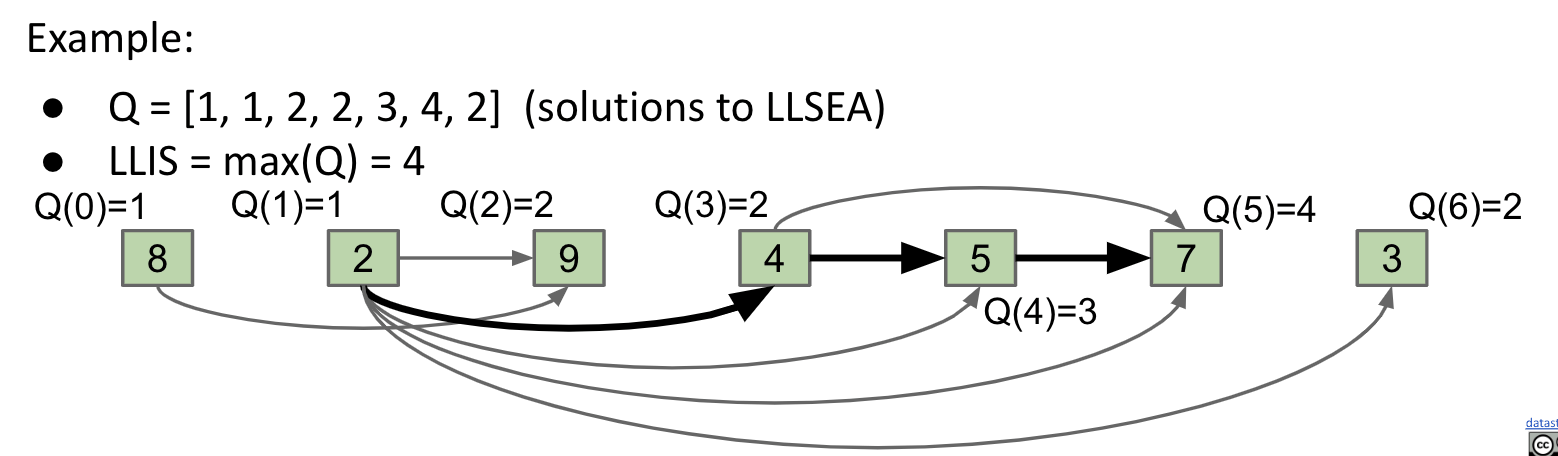#### DP Solution

Note: The slide’s solution has some bugs. $Q$ should be initialized with $1$, otherwise $Q[K] = Q[L] + 1$ would make $Q$ values $-\infty + 1$.

Setting them as $1$ is sensible since each number itself is already a 1-length increasing subsequence.

• Create $Q$, an array of length $N$. Set $Q = 1$, and $Q[K] = -\infty$ for rest of the vertices. (Check the Note above. It should be $1$)
• Create a DAG with $N$ vertices. Draw an edge between vertices if left vertex is less than right vertex. (Optional)

For each $K = 1,\ \ldots, N$:

• Then for each $L = 0,\ \ldots, K - 1$:
• If there exists an edge from $L$ to $K$: (or $L < K$)
• If $Q[L] + 1 > Q[K]$ (update when necessary)
• Set $Q[K] = Q[L] + 1$

Note: The DAG is not necessary. We can remove it and change the following:

• If there exists an edge from $L$ to $K$

to:

• If item $L$ is less than item $K$

Runtime:

Note: The runtimes are worst case.

• Initialization: Creating $Q$ array and DAG is $\Theta(N)$ and $\Theta(N^2)$ respectively.
• Execution: Nested for loops with constant time if statement take $\Theta(N^2)$.

#### Summary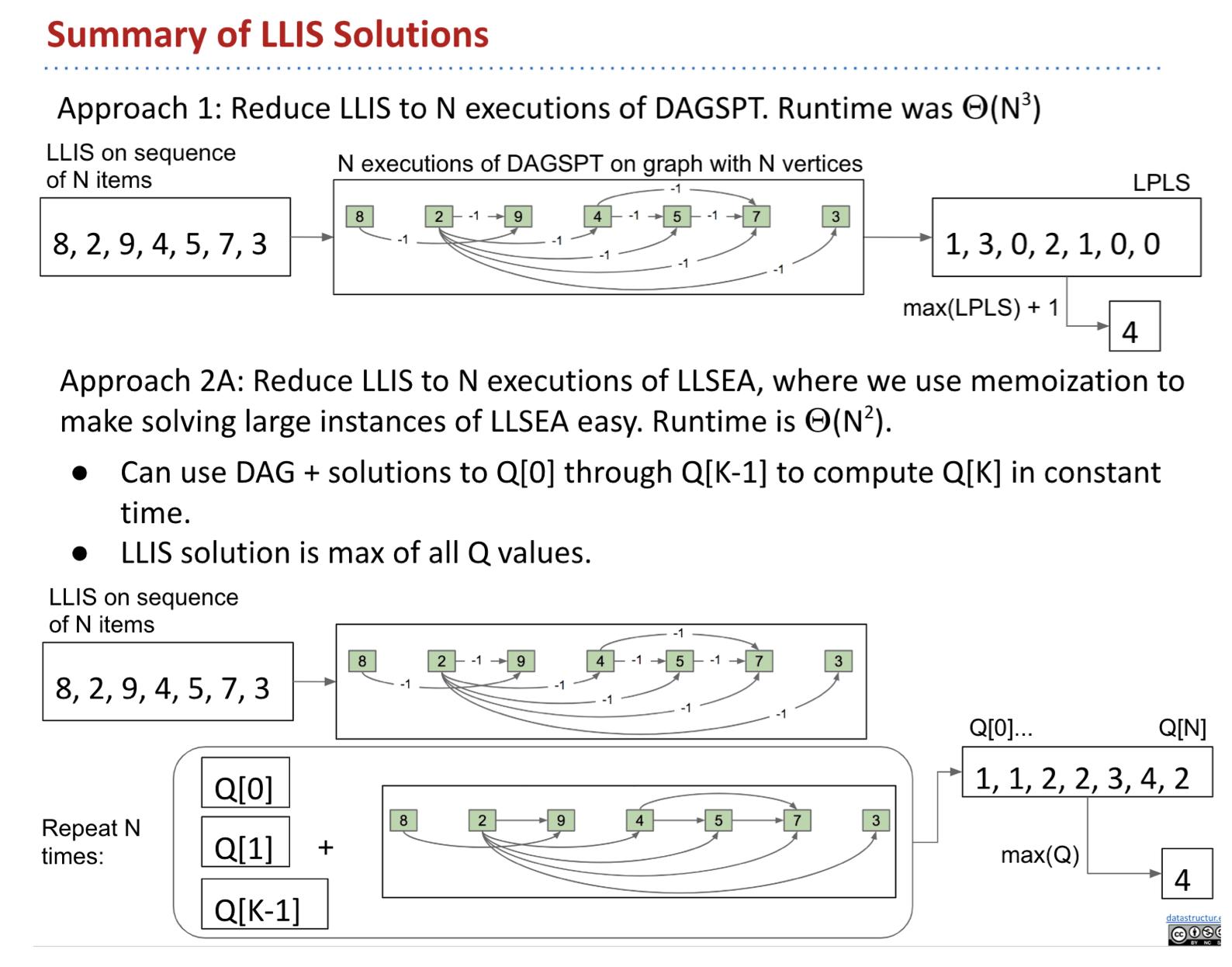## Independent Set Problem

Idea: 3SAT Problem can reduce to Independent Set Problem.

CommentJunhao Wang
a software engineering cat